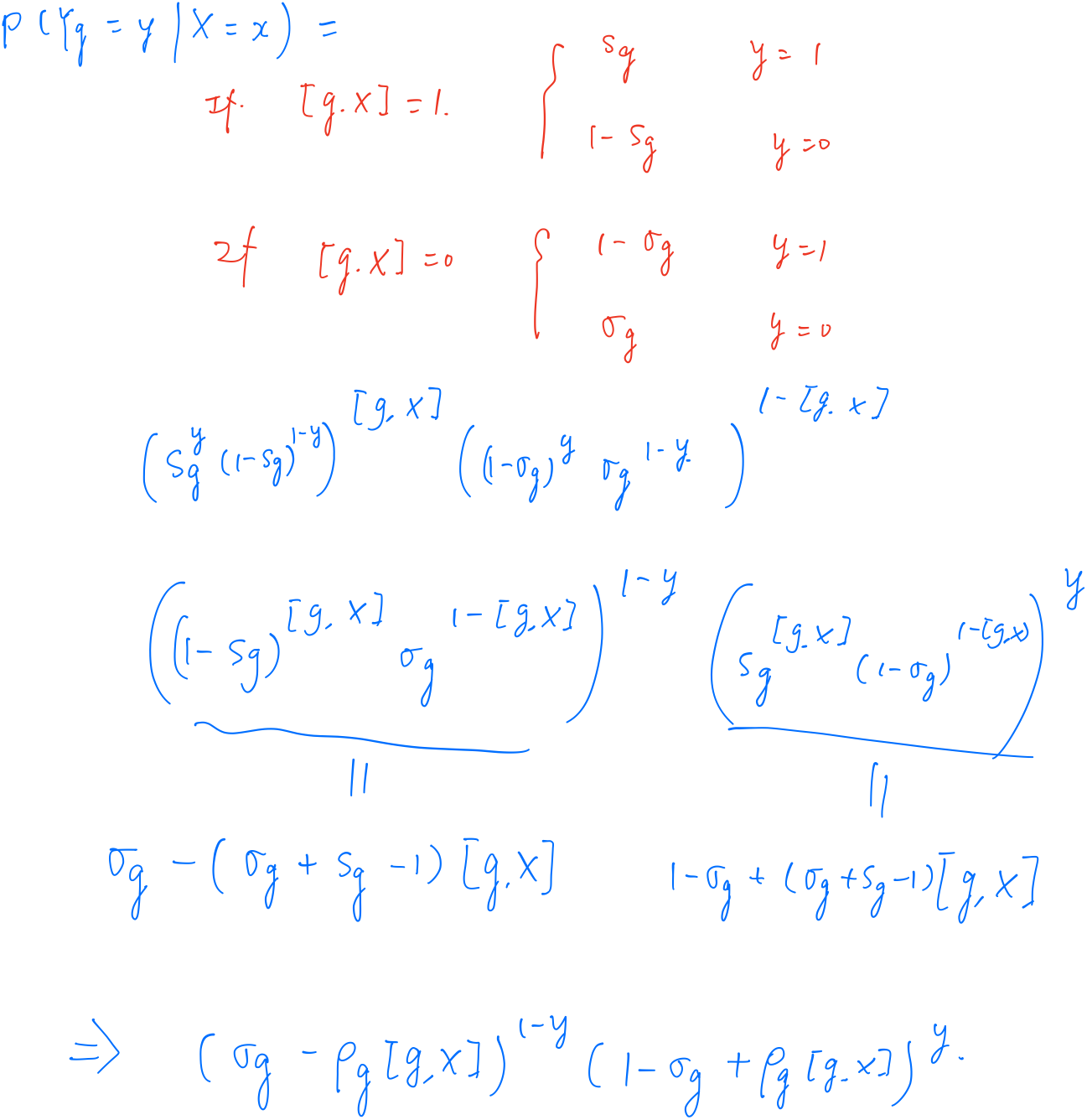# WeiYa's Work Yard

##### Posted on Jul 30, 2020

By Wikipedia, group testing is any procedure that breaks up the task of identifying certain objects into tests on groups of items, rather than on individual ones. First studied by Robert Dorfman in 1943.

Schemes for carrying out group testing:

• adaptive procedures: the tests for the next stage depend on the results of the previous stages
• non-adaptive procedures: all the tests are known beforehand

Goal of the paper: propose new group testing algorithms that can operate in a noisy setting (tests can be mistaken) to decide adaptively (looking at past results) which groups to test next, with the goal to converge to a good detection, as quickly, and with as few tests as possible.

Cast it as a Bayesian sequential experimental design problem: using the posterior distribution of infection status vector for $n$ patients, given observed tests carried out so far, seek to form groups that have a maximal utility. Practically, the posterior distributions on ${0, 1}^n$ are approximated by sequential Monte Carlo (SMC) samplers and the utility maximized by a greedy optimizer.

## Background on Group Testing

### Prior on infection

• $X_i$: the infection status of the $i$-th individual, $i=1,\ldots,n$
• $\bfX = (X_1,\ldots,X_n)\in {0, 1}^n$
• the prior may be independent Bernoulli, or other informed and non-independent priors. The paper approximated the prior $\pi_0$ with a weighted cloud of particles in ${0,1}^n$.

### Group vs individual testing in the presence of testing noise

two issues for testing individually

• costly when $n$ is large, and inefficient if infection prevalence is low
• noisy, so by testing only once each individual, there is a risk error. group testing no such problem, I mean, the group with negative results??

### Probabilistic inference from a single group

• $[n]:={1,\ldots,n}$
• $\calG$: set of all non-empty groups
• With a slight overload of notations, a group $\bfg$ is equivalently as a binary vector $\bfg\in {0,1}^n$.
• $g=\bfg^T\1$: the size of group $\bfg$
$\forall \bfg,\bfx \in \{0,1\}^n, [\bfg, \bfx]:= \bigvee_{i\in\bfg}x_i= 1-\prod_{i\in \bfg}(1-x_i) = \max(1, \bfg^T\bfx)\in \{0, 1\}\,,$
• $Y_\bfg$ assesses the group status $[\bfg,\bfX]$
• each group suffers from noise due to the specificity $\sigma_g$ and sensitivity $s_g$
$P(Y_\bfg=1\mid [\bfg, \bfX] = 1) = s_g, \qquad P(Y_\bfg=0\mid [\bfg, \bfX] = 0) = \sigma_g\,.$### Inference for batches of groups

assume that up to $k$ tests can be run simultaneously, in parallel, on a testing device. Propose a batch of $k$ groups $\bfG=(\bfg_1,\ldots,\bfg_k)\in \calG^k$, equivalently represented as a $n\times k$ binary membership matrix. Given a batch $\bfG$ of $k$ group, define the random vector $\bfY_\bfG:=(Y_{\bfg_1},\ldots,Y_{\bfg_k})$ of its $k$ independent test outcomes.

$P(\bfY_\bfG=\bfy\mid \bfX=\bfx) = \prod_{i=1}^k(\sigma_{g_i}-\rho_{g_i}[\bfg_i, \bfx])^{(1-y_i)}(1-\sigma_{g_i}+\rho_{g_i}[\bfg_i, \bfx])^{y_i}\,.$

## Bayesian Optimal Experimental Design to Select Useful Groups

Given a finite horizon $T\in \bbN$, the goal is to select sequentially batches of groups $\bfG^t\in\calG^k$ at each stage $1\le t\le T$. At the end of stage $t\ge 1$, batches $\bfG^1,\ldots,\bfG^t$ were selected previously, and tested with observed outcomes, $\bfY_{\bfG^1}=\bfy^1, \ldots, \bfY_{\bfG^t}=\bfy^t$.

Denote by $P_t$ the probability conditioned to all tests seen up to stage $t$, i.e., for any new batch $\bfG$,

$P_t(\bfX=\bfx, \bfY_\bfG=\bfy_\bfG):=P(\bfX=\bfx, \bfY_\bfG=\bfy_\bfG\mid \bfY_{\bfG^1}=\bfy^1,\ldots, \bfY_{\bfG^t}=\bfy^t) = \pi_t(\bfx)\times P(\bfY_\bfG=\bfy_\bfG\mid \bfX=\bfx)\,,$

where $\pi_t$ is the posterior pmf of the vector $\bfX$ of infection states, given all those test results revealed up to stage $t$, i.e.,

$\pi_t(\bfx):=P_t(\bfX=\bfx) = P(\bfX=\bfx\mid \bfY_{\bfG^1}=\bfy^1,\ldots,\bfY_{\bfG^t}=\bfy^t)$

### Maximizing mutual information

The mutual information (MI) utility is

$U_{MI}(\bfG, \pi_t):=I_{P_t}(\bfX; \bfY_\bfG) = H_{P_t}(\bfY_\bfG) - H_{P_t}(\bfY_\bfG\mid \bfX) = H_{P_t}(\bfY_G\mid \bfX)-\sum_\bfx\pi_t(\bfx)H_P(\bfY_\bfG\mid \bfX=\bfx)$

### Other utilities

Define $\pi_t^G(\bfx):=P_t(\bfX=\bfx\mid \bfY_\bfG)$, and choose

$\bfG^{t+1}\in \argmax_{\bfG\in\calG^k}U_\Phi(\bfG, \pi_t):=E_{P_t}\Phi(\pi_t^G)\,.$

With particular choice, it recovers the MI criterion. But it is used to defined the AUC-related quantity.

## Algorithms

• SMC sampler

Published in categories Note